A repeated series of actions and variables is a process. A collection of processes is a system. Virtually perfect Six Sigma quality results from an optimal interaction of all the variables in a given system.

Process and system questions we all face at work include: Which variables are the most important to the customer? Am I being efficient? Am I being effective? Am I using the best methods to complete my tasks? Is there a better way?

The science of data collection has two keys we can use to answer these questions. By systematically observing processes and systems, we learn faster than we do through trial and error. As Yogi Berra said; “You can see a lot just by looking.” When we look and learn, we can improve. Six Sigma counts, measures, and graphs speed learning.

### Key #1: Develop Crystal Clear Operational Definitions

Define precisely what you mean to count or measure before you start to count and measure. This is tricky business. For example, write down your definition of the word ‘pan’ on the following line.

____________________________________________________________

Good work. Your definition is correct. So are at least 10 others. In Spanish, pan means bread. Pan is a cooking container, a depression in the earth, a cavity in the lock of a flintlock, and the Greek god of the woods. You can pan a camera or pan for gold. Be very, very, specific when you define what it is that you intend to count or measure.

Here is another example of why clear operational definitions are crucial to even a simple process like counting. Count the number of f’s in the following paragraph.

FOR CENTURIES IMPORTANT PROJECTS HAVE BEEN DEFERRED BY WEEKS OF INDECISION AND MONTHS OF STUDY AND YEARS OF FORMAL DEBATE.

Depending on how you decided to define the letter “f” there are seven possible correct answers. There are no lower case f’s. So, zero is one correct answer. If you decided to count any F, there are 6. If you proof read phonetically, in other words you defined “f” by the sound of the letter, the F in each OF sounds like a “v.” So, if you defined an F by the way it sounds you could have counted 1, 2, 3, 4, 5 or 6. Any one, or all of these answers, taken in the context of its definition, could be considered to be correct.

### Key #2: Array Your Data in Columns and Rows

Tables are the proven way to array the data you collect in columns and rows. Data can be collected from any process.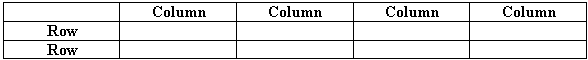In our computer age, operational definitions for ‘row’ and ‘column’ have changed. Rows are now called “Records.” Columns are “Fields.” Fields describe details about each record. Recording each record in its proper sequence is exceptionally important. Record the data sequence for every Six Sigma analysis. A four-field array is illustrated below.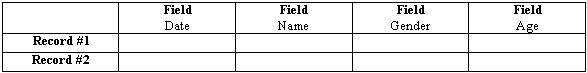This Six Sigma data array of fields and records would tell us a little about each observation. The more fields, the richer our understanding can be. For example, in the same amount of space the following table has twice as much data. Rich data, meaning each column/field has a crystal clear operational definition, can yield rich information. Many times we collect dozens of fields for each recorded observation. Since data collection is time consuming and expensive, design your collection plan with care before you begin.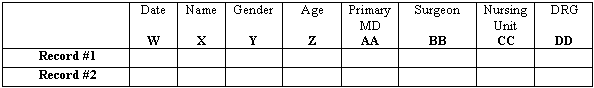Spreadsheet example: Excel spreadsheets help accounting and finance professionals scan vast amounts of data. Spreadsheets help them spot patterns. As helpful as this format is to their trained eyes, each record/row and column/field in a traditional spreadsheet mixes different fields and records together. This heterogeneous characteristic does not lend itself to a computer powered, statistically valid Six Sigma analysis.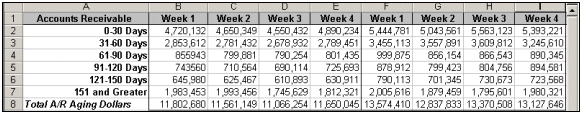Six Sigma array – spreadsheet example: The following Six Sigma, Six Sigma, array presents A/R aging dollars data in a way that leverages 21st Century computing power. Note the sequential time frame in the first column. The entire table goes back years, week-by-week, record-by-record. Each field is homogeneous. Uniform arrays promote the use of accurate, Six Sigma statistical analyses as well as improving eyeball assessments.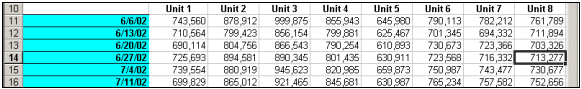### Four Rules For Data Analysis

Statistical methods deliver the highest level of evidence for making judgments. A statistical analysis is the surest way to confirm whether what we have observed is due to chance variations, or due to something special. Without an analysis, we have only intuition, assumptions, and guesses. Sometimes our assumptions are correct, sometimes not. Six Sigma reduces guesswork. Statistical analyses increase confidence in the judgments we must make in an uncertain world.

1. Calculate the average value for your set of numbers. A set of numbers is called a data set. The average, or mean, is symbolized in Six Sigma by the character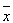. This symbol is pronounced X-bar. Excel’s paste function key (fx) can and will calculate averages automatically
2. Calculate the standard deviation,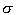, for your data set. The symbol for the standard deviation is called sigma,. Excel’s paste function key (fx) or other Six Sigma software can and will calculate this statistic automatically
3. Calculate the probability information for your data set. Probability information tells us if the differences we see in our counts or measures are due to random chance. Or, probability will tell us if the differences we see are most likely due to one factor or a combination of variables. Excel’s ANOVA (analysis of variation) function automatically calculates an essential probability statistic called an F ratio for you
4. Graph your data using an appropriate analytic graph. Excel’s bar graphs and pie charts are descriptive, not analytic. The affordable Six Sigma software add-ins illustrated later in this manual work seamlessly with Excel to graph the average, standard deviation, and probability information in more meaningful ways

Clearly, basic spreadsheet skills are an expected competency for Six Sigma executives who expect their Black Belt experts to produce best results. Computing power makes Six Sigma initiatives possible. Prioritize learning strategies to your personal needs.

When we turn our counts and measures into accurate statistical pictures, patterns emerge. These precious, time and money saving patterns would otherwise remain buried in columns and rows of numbers. Learning to recognize these patterns is an indispensable Six Sigma skill. Valuing the information conveyed by these patterns is one of the most important contributions executive leaders can make to Six Sigma projects.